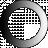# American Dictionary of the English Language

Dictionary Search

### Logarithm

LOG'ARITHM, noun [Gr. ratio, and number.]

Logarithms are the exponents of a series of powers and roots.

The logarithm of a number is that exponent of some other number, which renders the power of the latter, denoted by the exponent, equal to the former.

When the logarithms form a series in arithmetical progression, the corresponding natural numbers form a series in geometrical progression. Thus,

Logarithms

0 1 2 3 4 5

Natural numbers, 1 10 100 1000 10000 100000

The addition and subtraction of logarithms answer to the multiplication and division of their natural numbers. In like manner, involution is performed by multiplying the logarithm of any number by the number denoting the required power; and evolution, by dividing the logarithm by the number denoting the required root.

Logarithms are the invention of Baron Napier, lord of Marchiston in Scotland; but the kind now in use, were invented by Henry Briggs, professor of geometry in Gresham college at Oxford. They are extremely useful in abridging the labor of trigonometrical calculations.